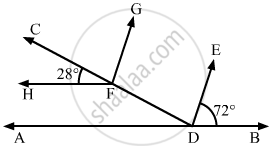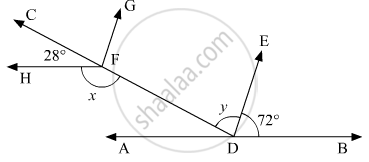# In the Given Figure, If Ab || Hf and De || Fg, Then the Measure of ∠Fde is - Mathematics

MCQ

In the given figure, if AB || HF and DE || FG, then the measure of ∠FDE is• 108°

• 80°

• 100°

• 90°

#### Solution

The given figure is as follows:It is given that . AB || HF

Thus, x and ∠HFC form a linear pair, therefore,

∠HFC + x = 180°

28° + = 180°

x = 180° - 28°

x = 152°       (1)

Also ED || GF

Thus, x and ∠FDB are corresponding interior opposite angles, therefore,

∠FDB = x

y + 72° = x

y = x - 72°

From (i):

y = 152° - 72°

y = 80°

Thus, ∠FDE = 80°

Concept: Pairs of Angles
Is there an error in this question or solution?
Chapter 10: Lines and Angles - Exercise 10.6 [Page 55]

#### APPEARS IN

RD Sharma Mathematics for Class 9
Chapter 10 Lines and Angles
Exercise 10.6 | Q 19 | Page 55

Share